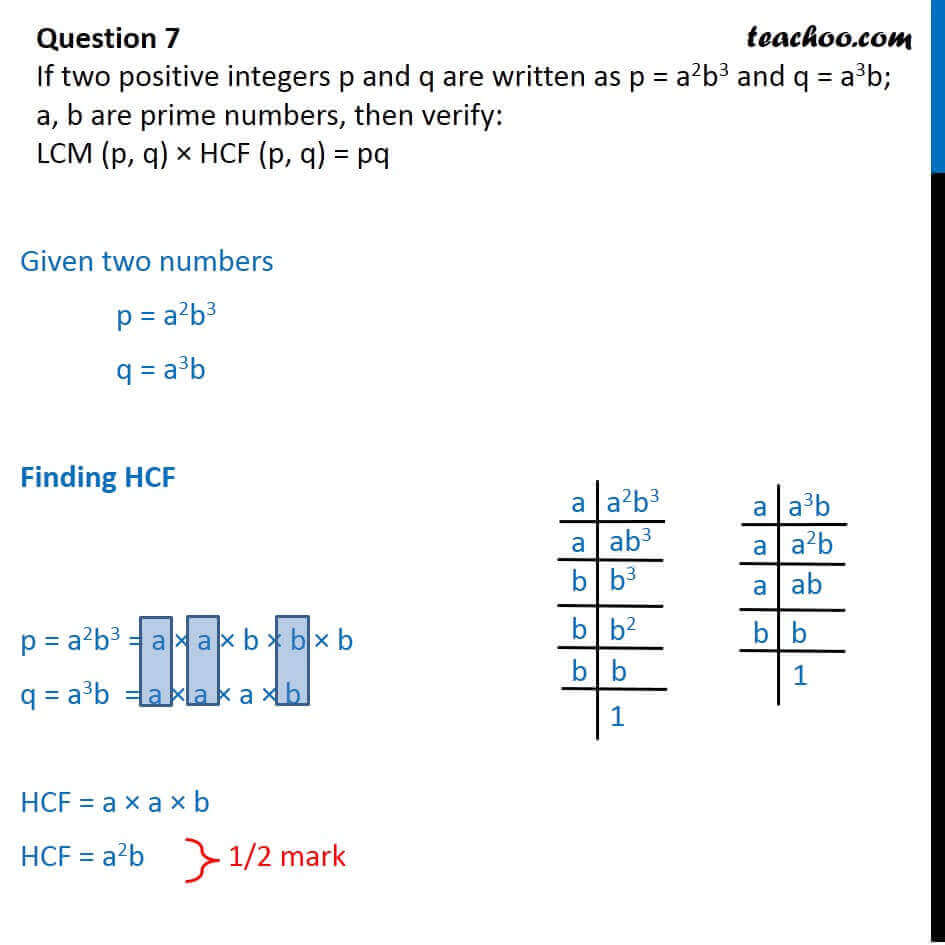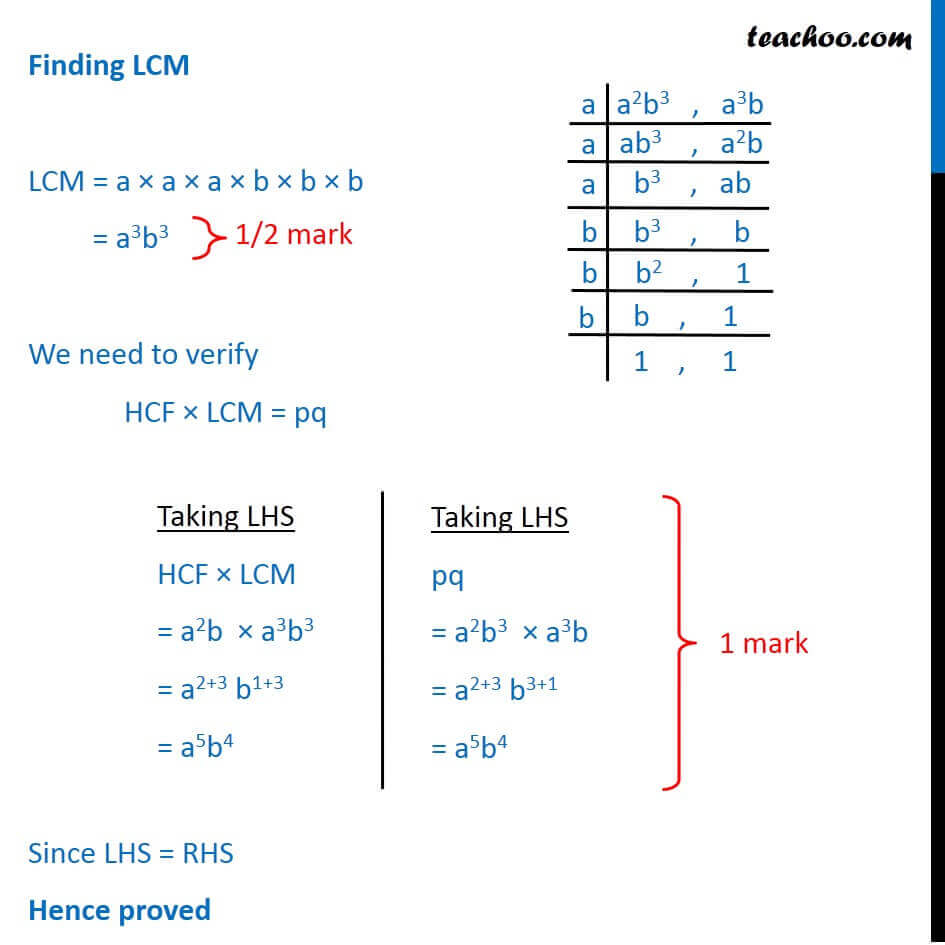CBSE Class 10 Sample Paper for 2018 Boards

Class 10
Solutions of Sample Papers for Class 10 Boards

### LCM (p, q) × HCF (p, q) = pq

This is a question of CBSE Sample Paper - Class 10 - 2017/18.Get live Maths 1-on-1 Classs - Class 6 to 12

### Transcript

Question 7 If two positive integers p and q are written as p = a2b3 and q = a3b; a, b are prime numbers, then verify: LCM (p, q) HCF (p, q) = pq Given two numbers p = a2b3 q = a3b Finding HCF p = a2b3 = a a b b b q = a3b = a a a b HCF = a a b HCF = a2b Finding LCM LCM = a a a b b b = a3b3 We need to verify HCF LCM = pq Since LHS = RHS Hence proved Taking LHS HCF LCM = a2b a3b3 = a2+3 b1+3 = a5b4 Taking LHS pq = a2b3 a3b = a2+3 b3+1 = a5b4